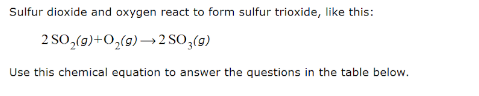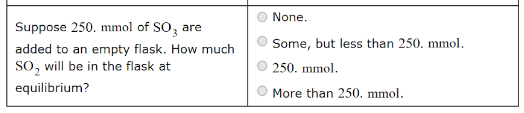# Sulfur dioxide and oxygen react to form sulfur trioxide, like this: 2 SO2(g) + O2(g) → 2 SO3(g) Use this chemical equation to answer the questions in the table below. Suppose 250. mmol of SO3 are added to an empty flask. How much SO2 will be in the flask at equilibrium?